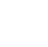Back to Chapter
Java IntroductionJava BasicsBranching and LoopingClasses, Objects, and MethodsArrays, Strings, and VectorsPackagesErrors and ExceptionApplet ProgrammingGraphics ProgrammingManaging I/O FilesJava Database Connectivity# Java Arrays

Let's consider a scenario, We want to store data items of similar types in a sequence, then the array is used. An array is a group of related data items that have a common name. It is a data structure where we store similar elements. We can store only a fixed set of elements in a Java array. Array index starts from 0. The main advantage of the array is Random access. We can get any data located at an index position.

# Types of Array in java

There are two types of array.

• Single Dimensional Array
• Two Dimensional Array

## 1.Single Dimensional Array:

A one-dimensional array (or single dimension array) is a type of linear array. Accessing its elements involves a single subscript that can either represent a row or column index.

Index:

Indexes are also called subscripts. An index maps the array value to a stored object. The first element of the array is indexed by a subscript of 0.

syntax:

type []arrayname; (or)
type arrayname[];

Instantiation of an Array:

An array is instantiated to create memory using new keyword.

arrayname = new type[size];

Initialization of Arrays:

arrayname[subscript] = value; // initialization of arrays

type arrayname[] = { list of values }; // declaration and initialization of arrays

Example:

class ArrayExample {
public static void main(String args[]) {
int a[] = new int; //declaration and instantiation
a = 10; //initialization
a = 20;
a = 30;
a = 40;
a = 50;
//traversing array
for (int i = 0; i < a.length; i++) //length is the property of array
System.out.println(a[i]);
}
}
/*OUTPUT:
10
20
30
40
50  */

## 2.Two Dimensional Array:

The two-dimensional array can be defined as an array of arrays with two subscripts. The two-dimensional array is organized as matrices which can be represented as the collection of rows and columns.

syntax:

type arrayname[rows][columns];

Instantiation of an Array:

An array is instantiated to create memory using new keyword.

arrayname = new type[row-size][column-size];

Initialization of Arrays:

arrayname[subscript][subscript] = value; // initialization of arrays

type arrayname[][] = { list of values }; // declaration and initialization of arrays

Example:

import java.lang.*;
class ArrayExample {
public static void main(String args[]) {
//declaring and initializing 2D array
int num[][] = {
{1,2,3},
{4,5,6},
{7,8,9}
};
//printing 2D array
for (int i = 0; i < 3; i++) {
for (int j = 0; j < 3; j++) {
System.out.print(num[i][j] + " ");
}
System.out.println();
}
}
}
/*OUTPUT:
1  2  3
4  5  6
7  8  9 */